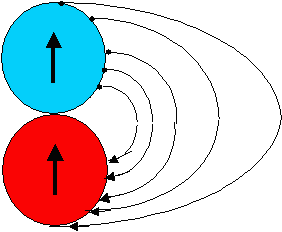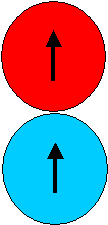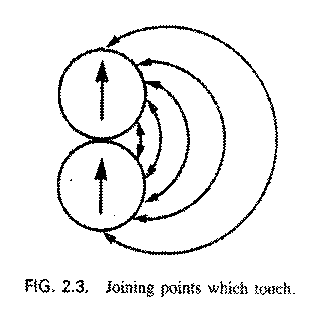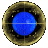Newtonian Differential Approach to Coin Dragon

The Differential Heuristic is a bundle of problem solving ideas based upon the following scheme: Track the motion Step By Tiny Step. The heuristic Differential is applied to systems in which there is motion, and involves looking point-by-point at each instant of motion, and working out in (sufficient) detail what's going on there_and_then. This heuristic more than any other involves the Newtonian viewpoint that is emphasised in physics courses.
 To apply the Differential strategy, we need to trace out in detail the motion of the blue coin as it rolls -- without slipping -- around the red. This we have done in the adjacent diagram, where we have shown where points on the top (blue) coin -- marked with a blob -- touch the lower (red) coin during the rolling. When the blue coin has through half its circumference along half the circumference of the red coin, then the top of blue coin is touching the bottom of the red coin, like so:Note that the top of the blue coin ends up touching the bottom of the red coin -- meaning that the blue coin rolls until finallyThe same Differential motivated approach is implicit in the adjacent diagram:: which shows, point by point, which circumferential points are touching during the "real" rolling. This figure is reproduced from the paper, Andrea di Sessa, "Phenomenology and the Evolution of Intuition". in Deidre Gentner and Albert L. Stevens, (Editors), Mental Models, Lawrence Erlbaum Assoc., Hillsdale N.J., pp 15-33 (1983).Physics postgraduate students and professors often present this solution, they are so readily cued-in to apply Newton's differential heuristic whenever there is motion. But other heuristics are very applicable to the "Coin Problem", even one that relates muchly to a very well-noted aspect of Einstein's physics. See Albert's solution, and others, from this choice:Process motivated solution to the Coin ProblemReciprocity/Symmetry solution to the Coin ProblemEinstein's solution to the Coin ProblemHomepage for the Coin ProblemReturn To Dragons Homepage